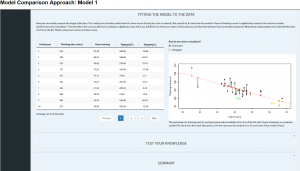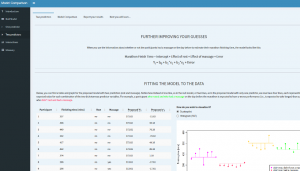With this app you can explore basic statistics concepts, compare different models and see how they all boil down to the same recipe:

Data = model + error

### Model Comparison Approach: Model 1

Model 1 uses one metric independent variable and a metric dependent variable and offers a basic introduction of the model comparison approach and an introduction to model comparison using linear regressions and correlations.### Model Comparison Approach: Model 2

Model 2 uses one categorical independent variable and a metric dependent variable and offers a more advanced discussion using linear regressions with dummies, t-test, and factorial ANOVA.